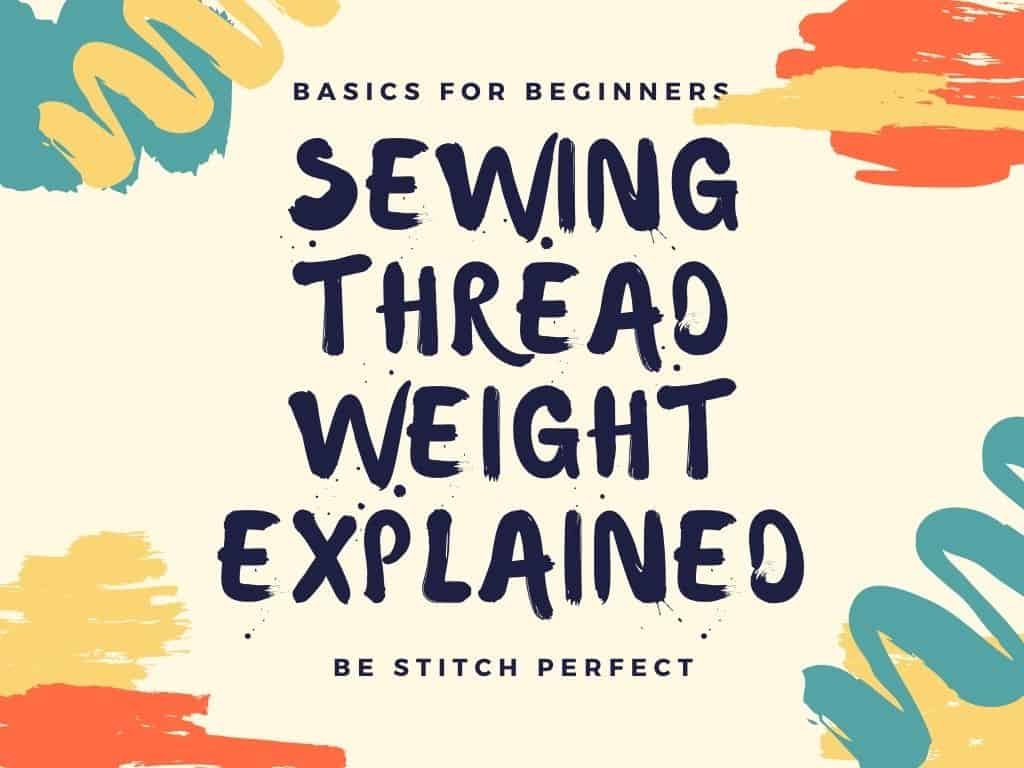Sewing thread, no matter what material it may be made from, comes in different ‘weights’. But what is thread weight, how is it measured, what does it mean, and why might we want to understand thread weight? This article will cover everything you need to know about thread weight and why it is important.

## Why do we need to understand thread weight?

Using the correct thread for your project is critical to the final outcome. I’ll grant you that most of the time, using a ‘standard’ thread is appropriate for a lot of projects so you could just sew away and not worry about it for years, and most of the time things would work out just fine.

But have you ever had problems with thread breaking while you sew, skipped stitches, or the thread ‘shredding’ and getting thin? Incorrect thread weight could be the issue. Or a mis-match of thread weight to needle size. We’ll cover more about sewing needles and everything you need to know there in another article, but for now, let’s concentrate on learning more about the thread.

## How is thread weight measured?

Well, this is a bit of a trick question because there seems to be no one universal way to ‘weigh’ thread or give it a thread weight. Basically, its a number derived from comparing the thread’s length and weight, but there are still several systems for measuring.

• English Count (Ne) = Number of hanks of 840 yards per lb
• Metric Count (Nm) = Number of hanks of 1000 metres per kg
• Denier = Weight in grams of 9,000 metres
• Tex = Weight in grams of 1,000 metres
• Decitex = Weight in grams of 10,000 metres

The English Count and the Metric Count would be most commonly used for sewing thread. Generally, Metric count is used to describe synthetic, spun and corespun thread while English count is used to specify cotton thread.

## Comparing thread weights – which is thicker?

So now you know that thread weight refers to a comparison between length and weight, you can work out which thread is thicker.

Using the Metric Count, a 40wt thread of 40km long weighs 1 kg. And a 28wt thread must therefore be 28km long to weigh 1 kg.

Therefore you need LESS of the 28wt thread to weigh the same amount. So for that to be true, it must be heavier, therefore thicker.

The SMALLER the number, the HEAVIER or THICKER the thread. So a 28wt thread is thicker than a 40wt thread. The 40wt would be a general purpose thread ideal for sewing up the body of your bag. The 28wt thread would be ideal for top stitching where you wanted a bolder look.

## What’s the second number refer to in thread weight?

Thankfully the second number is much easier to understand. If you see 40/2 or 28/3 for example, the first number is the ‘weight’ as described above and the second number is the ‘ply’. This tells you how many strands, or ‘plies’ of thread have been twisted together to make that thread. The more plies there are, the stronger the thread.